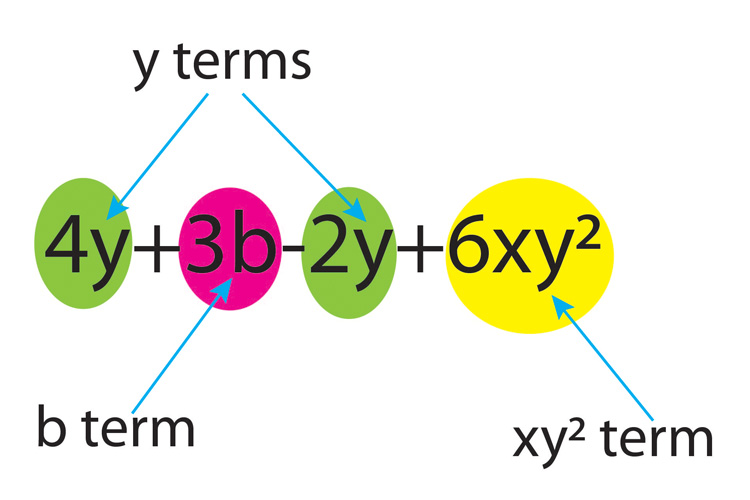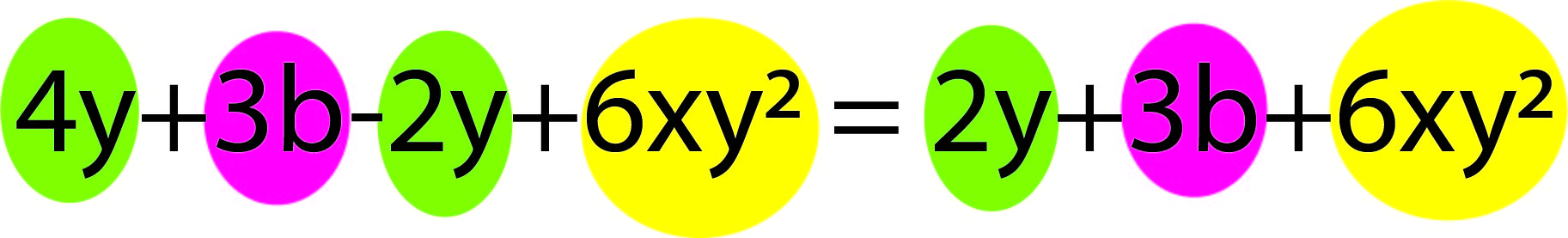# Like terms

Like terms are terms that contain the same series of letters and indices. Like terms in an equation may be added or subtracted from each other to simplify the equation.The y terms in the above equation can be combined to simplify the equation.Example 1

Simplify the following expression 3x^2+y^3-x^2-y^3

Note: By simplify they mean combine terms

3x^2+y^3-x^2-y^3

3x^2-x^2+y^3-y^3

2x^2+0

2x^2

Answer: 3x^2+y^3-x^2-y^3=2x^2

Example 2

Simplify the following expression 3xy-4ac+5yx+4ac

Note: By simplify they mean combine terms

3xy-4ac+5yx+4ac

3xy+5yx+4ac-4ac

3xy+5yx+0

Note: 5yx=5timesytimesx=5timesxtimesy=5xy

3xy+5xy

8xy

Answer: 3xy-4ac+5yx+4ac=8xy

Example 3

Simplify the following expression 2x^2+3x-5x^2-x+8

Note: By simplify they mean combine terms

2x^2+3x-5x^2-x+8

2x^2-5x^2+3x-x+8

-3x^2+2x+8

Answer: 2x^2+3x-5x^2-x+8=-3x^2+2x+8

Example 4

Expand and simplify the following 2x^2(4xy-5)-8yx^3+9x^2

Note: By simplify they mean combine terms

2x^2(4xy-5)-8yx^3+9x^2

8x^3y-10x^2-8yx^3+9x^2

8x^3y-8yx^3+9x^2-10x^2

cancel(8x^3y)-cancel(8yx^3)+9x^2-10x^2

Answer: 2x^2(4xy-5)-8yx^3+9x^2=-x^2